Divisible

Chapter 4 Class 11 Mathematical Induction
Concept wise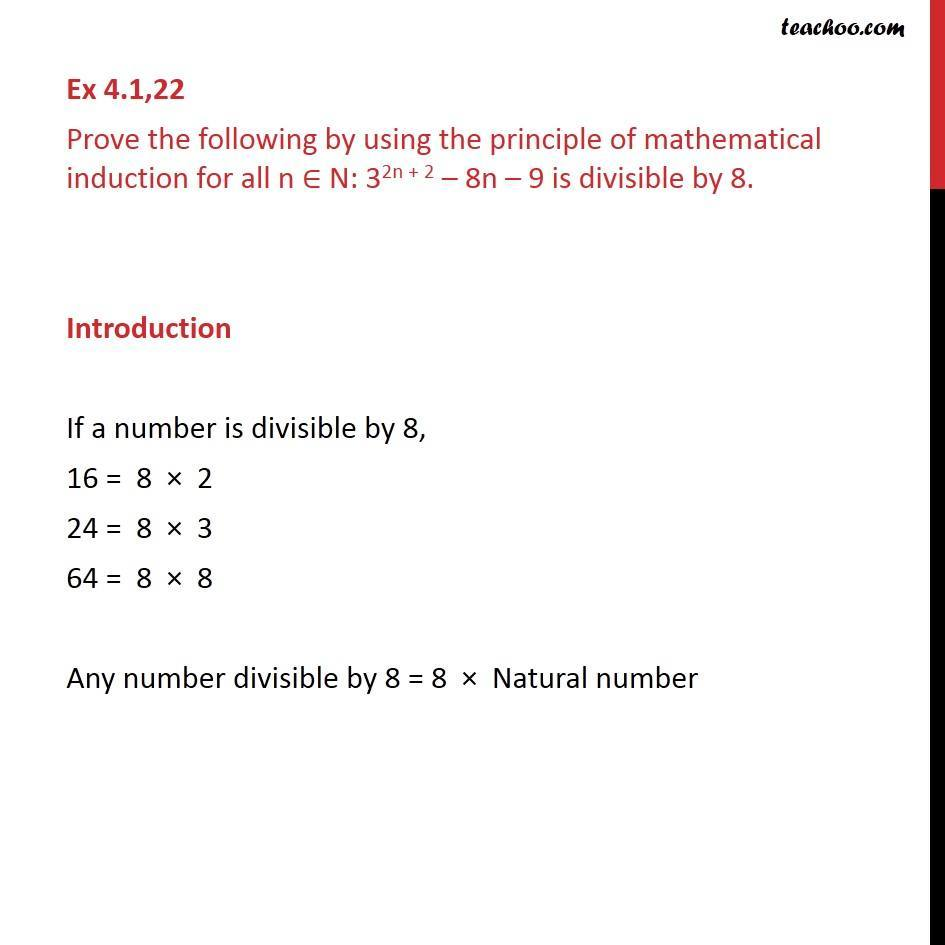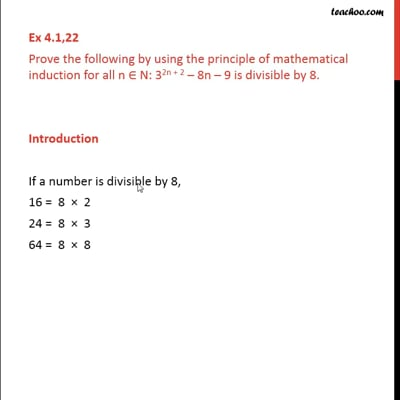This video is only available for Teachoo black users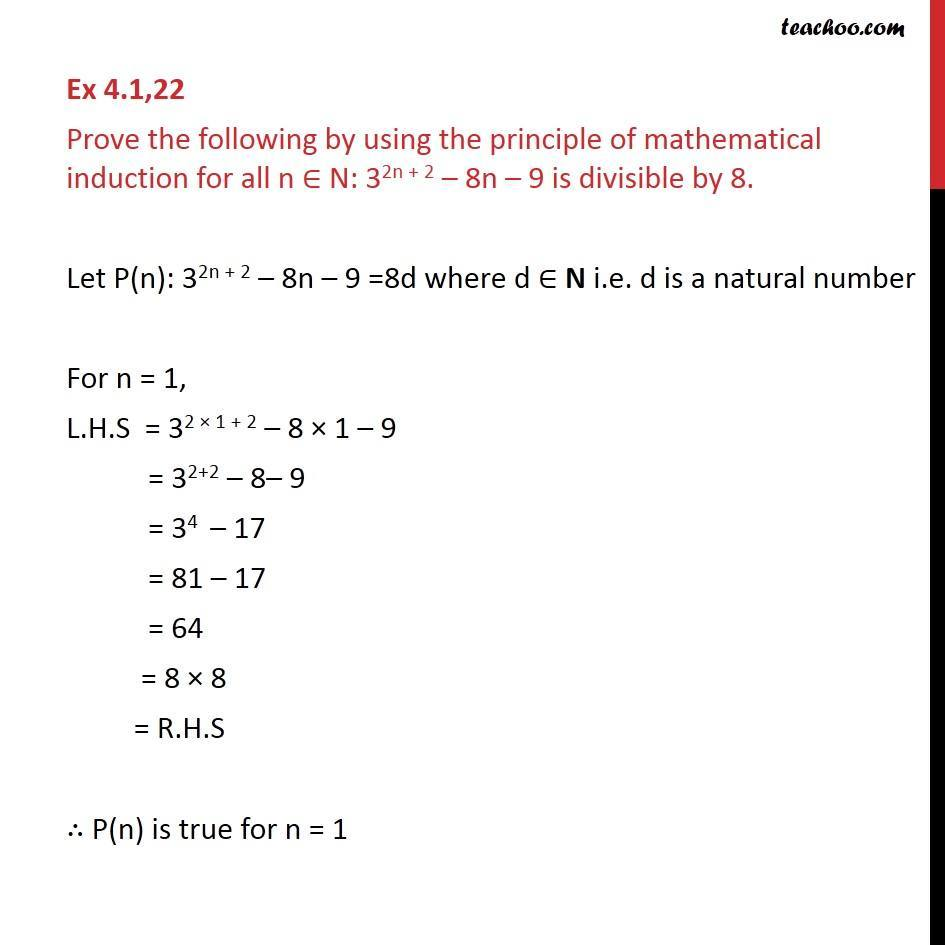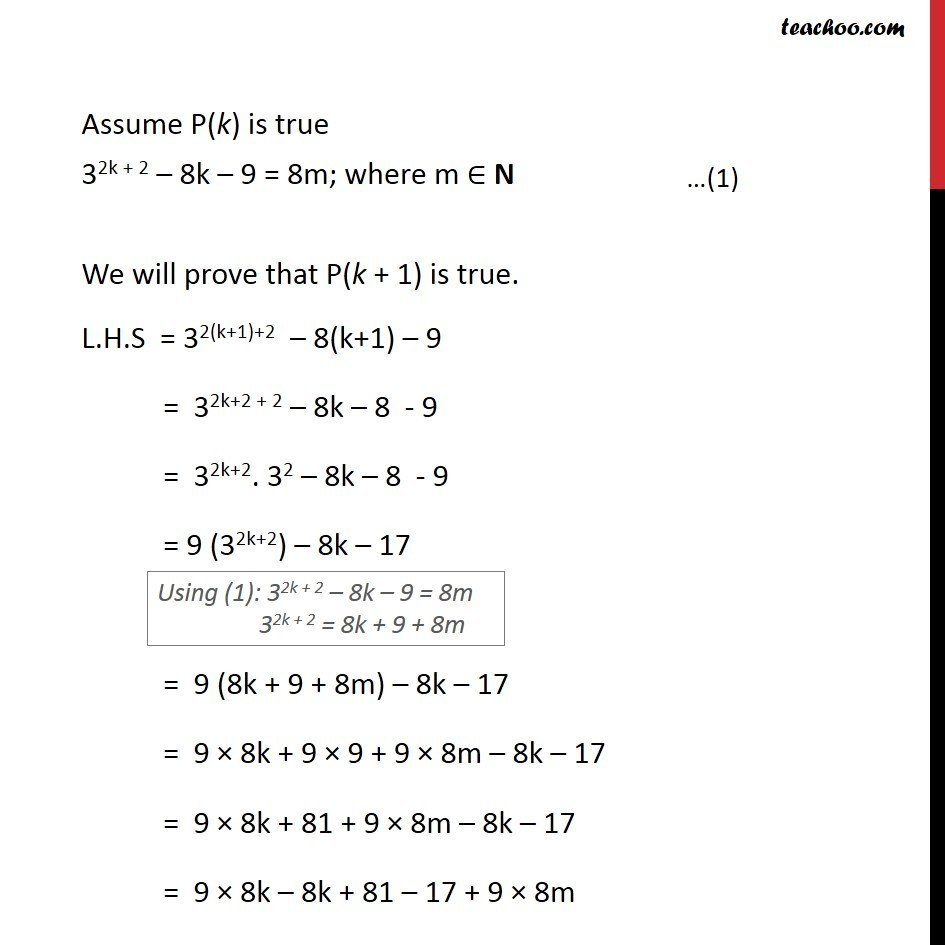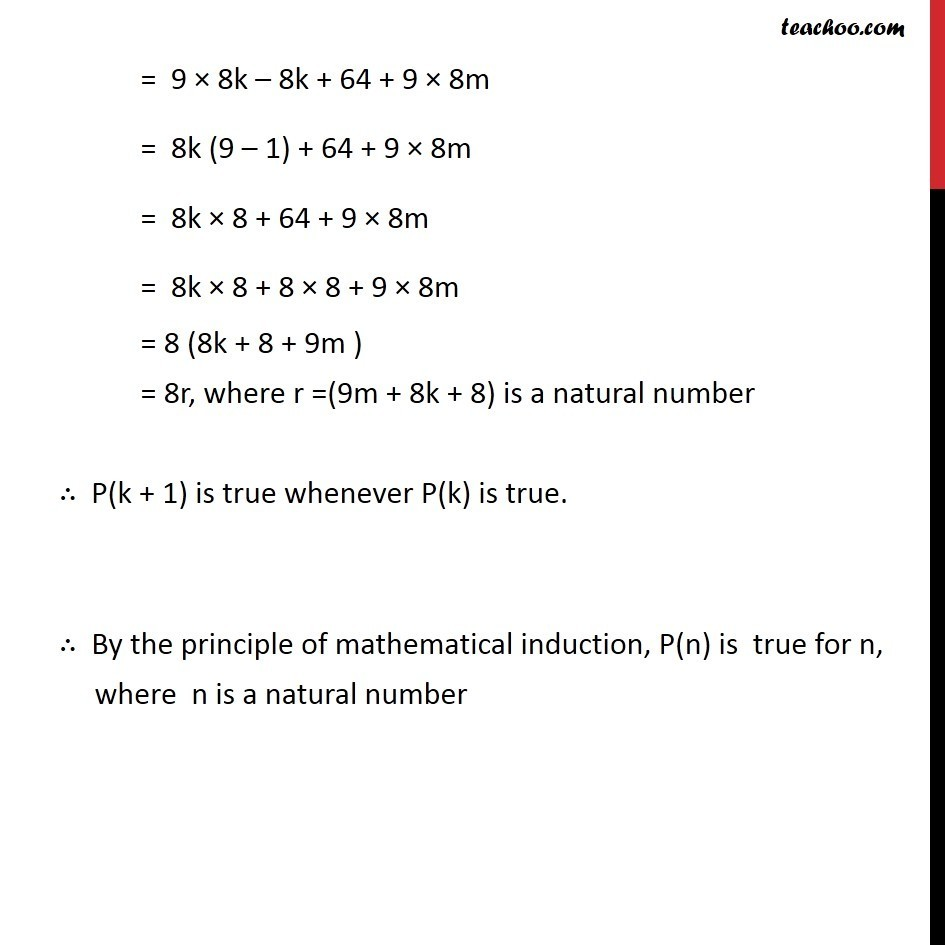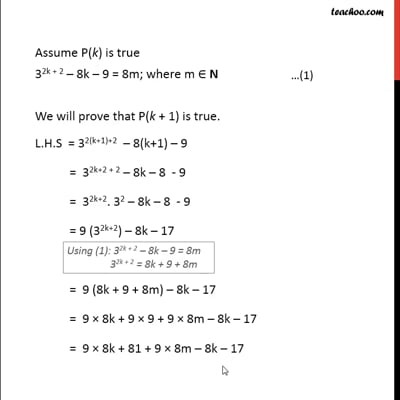This video is only available for Teachoo black users

Introducing your new favourite teacher - Teachoo Black, at only ₹83 per month

### Transcript

Ex 4.1,22 Prove the following by using the principle of mathematical induction for all n N: 32n + 2 8n 9 is divisible by 8. Introduction If a number is divisible by 8, 16 = 8 2 24 = 8 3 64 = 8 8 Any number divisible by 8 = 8 Natural number Ex 4.1,22 Prove the following by using the principle of mathematical induction for all n N: 32n + 2 8n 9 is divisible by 8. Let P(n): 32n + 2 8n 9 =8d where d N i.e. d is a natural number For n = 1, L.H.S = 32 1 + 2 8 1 9 = 32+2 8 9 = 34 17 = 81 17 = 64 = 8 8 = R.H.S P(n) is true for n = 1 Assume P(k) is true 32k + 2 8k 9 = 8m; where m N We will prove that P(k + 1) is true. L.H.S = 32(k+1)+2 8(k+1) 9 = 32k+2 + 2 8k 8 - 9 = 32k+2. 32 8k 8 - 9 = 9 (32k+2) 8k 17 = 9 (8k + 9 + 8m) 8k 17 = 9 8k + 9 9 + 9 8m 8k 17 = 9 8k + 81 + 9 8m 8k 17 = 9 8k 8k + 81 17 + 9 8m = 9 8k 8k + 64 + 9 8m = 8k (9 1) + 64 + 9 8m = 8k 8 + 64 + 9 8m = 8k 8 + 8 8 + 9 8m = 8 (8k + 8 + 9m ) = 8r, where r =(9m + 8k + 8) is a natural number P(k + 1) is true whenever P(k) is true. By the principle of mathematical induction, P(n) is true for n, where n is a natural number